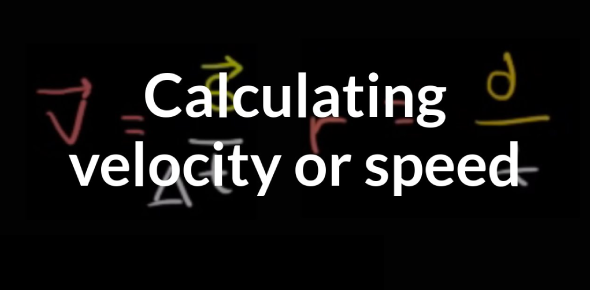Velocity And Speed! Ultimate Trivia Quiz

10 Questions | Total Attempts: 2109SettingsWhat do you know about velocity and speed? Velocity is the direction in which the object is moving, and it is the rate that the object is changing its position. Speed is the point in time and rate at which an item is moving along a path. In other words, a car can move at a speed of 65 miles per hour. If the vehicle veers out of its current path, that is velocity, and if you want to learn more, try this quiz.

• 1.
______ is a unit of speed:
• A.

M/s

• B.

S

• C.

Kg

• D.

Hr

• 2.
The speed at any instant of time is known as
• A.

Average speed

• B.

Velocity

• C.

Given speed

• D.

Instantaneous speed

• 3.
If a total distance of 750 m is covered in a time interval of 25 s, the average speed is ______?
• A.

3,974 mph

• B.

3 mph

• C.

30 mph

• D.

30 m/s

• 4.
______________ describes how fast something is going, whereas, __________ describes how fast something is going and in a certain direction.
• A.

Speed, velocity

• B.

Rate, speed

• C.

Rate, velocity

• D.

Speed, acceleration

• 5.
Which of the following is a measure of velocity?
• A.

30 s

• B.

30 South

• C.

30 m/s

• D.

30 m/s, South

• 6.
If a person walking at 2 m/s for 12 s he/she would travel a distance of ________.
• A.

24 m

• B.

6 m

• C.

4 m

• D.

• 7.
How long would it take to travel 50 km traveling at a speed of 10 km/hr?
• A.

3 hours

• B.

1 hour

• C.

5 hours

• D.

50 hours

• 8.
The ______ of a distance versus time graph is speed.
• A.

Slope

• B.

Y-intercept

• C.

Origin

• D.

• 9.
SI unit for speed is the ________.
• A.

In/ hour

• B.

M/s

• C.

Cm/min

• D.

• 10.
How far would you travel moving at 12 m/s for 3.00 minutes?
• A.

36.0 m

• B.

2160 m

• C.

40.0 m

• D.

36.0 miles

Related TopicsBack to top